# Common Core: High School - Number and Quantity : Complex Conjugate: CCSS.Math.Content.HSN-CN.A.3

## Example Questions

← Previous 1

### Example Question #1 : Complex Conjugate: Ccss.Math.Content.Hsn Cn.A.3

Find the product of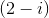and its complex conjugate.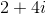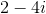Explanation:

This question tests one's understanding on complex numbers and their corresponding conjugate. It is important to remember that when a complex number is multiplied by its conjugate, the imaginary numbers disappear. A complex number and its conjugate takes the form as follows: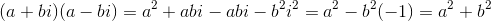Note: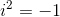For the purpose of Common Core Standards, "find the conjugate of a complex number; use conjugates to find moduli and quotients of complex numbers", falls within the Cluster A of "perform arithmetic operations with complex numbers" (CCSS.MATH.CONTENT.HSF.CN.A).

Knowing the standard and the concept for which it relates to, we can now do the step-by-step process to solve the problem in question.

Step 1: Using the formula above find the conjugate.

The formula states to keep the real portion and the imaginary portion but switch the sign between them.

Complex number:Real portion:Imaginary portion:Conjugate: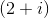Step 2: Set up the multiplication problem between the complex number and its conjugate.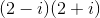Step 3: Multiply the two binomials together.

Distribute the first term in the first binomial to both terms in the second binomial. Then distribute the second term in the first binomial to each term in the second binomial.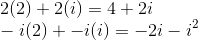From here combine the two new expressions together.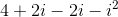Sincethe product becomes,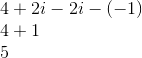### Example Question #1 : Complex Conjugate: Ccss.Math.Content.Hsn Cn.A.3

Find the conjugate of the complex number.Explanation:

2.

### Example Question #1 : Complex Conjugate: Ccss.Math.Content.Hsn Cn.A.3

Find the conjugate of the complex number.Explanation:

3.

### Example Question #1 : Complex Conjugate: Ccss.Math.Content.Hsn Cn.A.3

Find the conjugate of the complex number.Explanation:

4.

### Example Question #72 : High School: Number And Quantity

Find the conjugate of the complex number.Explanation:

5.

### Example Question #1 : Complex Conjugate: Ccss.Math.Content.Hsn Cn.A.3

Find the product of the complex number and its conjugate.Explanation:### Example Question #3 : Complex Conjugate: Ccss.Math.Content.Hsn Cn.A.3

Find the product of the complex number and its conjugate.Explanation:### Example Question #4 : Complex Conjugate: Ccss.Math.Content.Hsn Cn.A.3

Find the product of the complex number and its conjugate.Explanation:### Example Question #2 : Complex Conjugate: Ccss.Math.Content.Hsn Cn.A.3

Find the product of the complex number and its conjugate.Explanation:### Example Question #6 : Complex Conjugate: Ccss.Math.Content.Hsn Cn.A.3

Simplify.Explanation:← Previous 1

### All Common Core: High School - Number and Quantity Resources